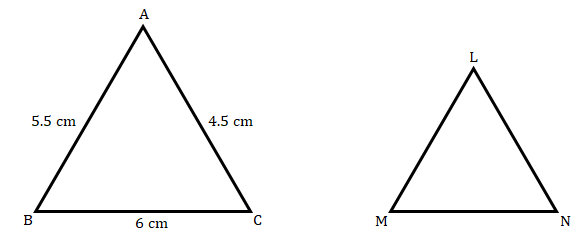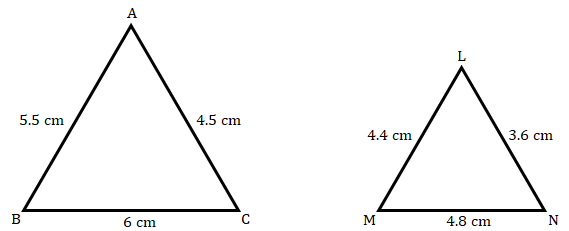# ГўЛҶвҖ ABC ~ ГўЛҶвҖ LMN. In ГўЛҶвҖ ABC, AB = 5.5 cm, BC = 6 cm, CA = 4.5 cm. Construct ГўЛҶвҖ ABC and ГўЛҶвҖ LMN such that $\frac{BC}{MN} \ =\ \frac{5}{4}$.

Given:

∆ABC ~ ∆LMN  and

$\frac{BC}{MN} \ =\ \frac{5}{4}$    ....(i)To find:

We need to Construct ∆ABC and ∆LMN.

Solution:

Now, we know that:

Corresponding sides of similar triangles are proportional. So,

$\frac{AB}{LM} \ =\ \frac{BC}{MN} \ =\ \frac{AC}{LN}$   ....(ii)

From equation (i) and (ii):

$\frac{AB}{LM} \ =\ \frac{BC}{MN} \ =\ \frac{AC}{LN} \ =\ \frac{5}{4}$   ...(iii)

Therefore,

$\begin{array}{l} \frac{AB}{LM} \ =\ \frac{5}{4}\ \ \ LM\ =\ AB\ \times \ \frac{4}{5}\ \ \ LM\ =\ 5.5\ \times \ \frac{4}{5}\ \ \ LM\ =\ 4.4\ cm \end{array}$

Also,

$\begin{array}{l} \frac{BC}{MN} \ =\ \frac{5}{4}\ \ \ MN\ =\ BC\ \times \ \frac{4}{5}\ \ \ MN\ =\ 6\ \times \ \frac{4}{5}\ \ \ MN\ =\ 4.8\ cm \end{array}$

And,

$\begin{array}{l} \frac{AC}{LN} \ =\ \frac{5}{4}\ \ \ LN\ =\ AC\ \times \ \frac{4}{5}\ \ \ LN\ =\ 4.5\ \times \ \frac{4}{5}\ \ \ LN\ =\ 3.6\ cm \end{array}$

So, the required triangles are as follows:Updated on: 10-Oct-2022

24 Views